Updating search results...

# 72 Results

View
Selected filters:
• Algebra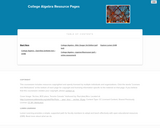Educational Use
Rating
0.0 stars

This course covers relations and functions, specifically, linear, polynomial, exponential, logarithmic, and rational functions. Additionally, sections on conics, systems of equations and matrices and sequences are also available.

Subject:
Algebra
Mathematics
Material Type:
Full Course
Textbook
Provider:
Lumen Learning
Provider Set:
Candela Courseware
Author:
Carl Stitz
Jeff Zeager
04/25/2019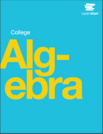Unrestricted Use
CC BY
Rating
0.0 stars

College Algebra provides a comprehensive and multi-layered exploration of algebraic principles. The text is suitable for a typical introductory Algebra course, and was developed to be used flexibly. The modular approach and the richness of content ensures that the book meets the needs of a variety of programs.College Algebraguides and supports students with differing levels of preparation and experience with mathematics. Ideas are presented as clearly as possible, and progress to more complex understandings with considerable reinforcement along the way. A wealth of examples – usually several dozen per chapter – offer detailed, conceptual explanations, in order to build in students a strong, cumulative foundation in the material before asking them to apply what they've learned.

OpenStax College has compiled many resources for faculty and students, from faculty-only content to interactive homework and study guides.

Access also available here: https://openstax.org/details/books/college-algebra

1 Prerequisites
2 Equations and Inequalities
3 Functions
4 Linear Functions
5 Polynomial and Rational Functions
6 Exponential and Logarithmic Functions
7 Systems of Equations and Inequalities
8 Analytic Geometry
9 Sequences, Probability, and Counting Theory

Subject:
Algebra
Mathematics
Trigonometry
Material Type:
Textbook
Provider:
Rice University
Provider Set:
OpenStax College
Author:
David Lippman
Jay Abramson
Jean-Marie Magnier
Melonie Rasmussen
Nicholas Belloit
Rachael Gross
Rick Norwood
Valeree Falduto
01/29/2015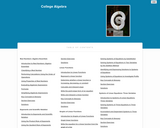Educational Use
Rating
0.0 stars

It is often said that mathematics is the language of science. If this is true, then the language of mathematics is numbers. The earliest use of numbers occurred 100 centuries ago in the Middle East to count, or enumerate items. Farmers, cattlemen, and tradesmen used tokens, stones, or markers to signify a single quantitya sheaf of grain, a head of livestock, or a fixed length of cloth, for example. Doing so made commerce possible, leading to improved communications and the spread of civilization.

Subject:
Algebra
Mathematics
Material Type:
Full Course
Textbook
Provider:
Lumen Learning
Provider Set:
Candela Courseware
04/25/2019Only Sharing Permitted
CC BY-NC-ND
Rating
0.0 stars

This course is designed to take the concepts you learn in developmental math to expand your knowledge of algebra. This course will focus on two major algebraic concepts to learn - how to SOLVE equations and how to GRAPH equations. Throughout this course you will be challenged to recall ALL of your prior knowledge of operations of real numbers as well as your knowledge related to solving and graphing linear equations (which you should have already mastered from developmental algebra). You will use this prior knowledge to expand on learning the following objectives: solving linear & rational equations. operations of complex numbers, solving quadratic equations, solving radical & polynomial equations, solving equations with rational exponents, solving linear and compound inequalities, solving absolute value equations and inequalities, graphing linear equations & slope, understanding concepts of domain, range and function notation, finding compositions of functions, finding inverses of functions, solving and graphing exponential and logarithmic equations, solving and graphing systems of equations and inequalities, and graphing conics.

*Open Campus courses are non-credit tutorials and cannot, in and of themselves, be used to satisfy degree requirements at Bossier Parish Community College (BPCC). (College Algebra Course by Bossier Parish Community College is licensed under a Creative Commons Attribution-NonCommercial-NoDerivatives 4.0 International License. Based on a work at http://bpcc.edu/opencampus/index.html.)

Subject:
Algebra
Mathematics
Material Type:
Full Course
Provider:
Bossier Parish Community College
Author:
Stacey Black
07/14/2020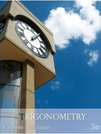Conditional Remix & Share Permitted
CC BY-NC-SA
Rating
0.0 stars

This book was designed as an introductory trigonometry textbook for college students with the explicit goal of reducing textbook costs.

Subject:
Algebra
Mathematics
Trigonometry
Material Type:
Textbook
Provider:
Stitz-Zeager Open Source Mathematics
Author:
Carl Stitz
Jeff Zeager
07/15/2011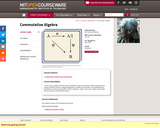Conditional Remix & Share Permitted
CC BY-NC-SA
Rating
0.0 stars

In this course students will learn about Noetherian rings and modules, Hilbert basis theorem, Cayley-Hamilton theorem, integral dependence, Noether normalization, the Nullstellensatz, localization, primary decomposition, DVRs, filtrations, length, Artin rings, Hilbert polynomials, tensor products, and dimension theory.

Subject:
Algebra
Mathematics
Material Type:
Full Course
Provider:
M.I.T.
Provider Set:
M.I.T. OpenCourseWare
Author:
Kleiman, Steven
01/01/2008Only Sharing Permitted
CC BY-NC-ND
Rating
0.0 stars

All of the mathematics required beyond basic calculus is developed “from scratch.” Moreover, the book generally alternates between “theory” and “applications”: one or two chapters on a particular set of purely mathematical concepts are followed by one or two chapters on algorithms and applications; the mathematics provides the theoretical underpinnings for the applications, while the applications both motivate and illustrate the mathematics. Of course, this dichotomy between theory and applications is not perfectly maintained: the chapters that focus mainly on applications include the development of some of the mathematics that is specific to a particular application, and very occasionally, some of the chapters that focus mainly on mathematics include a discussion of related algorithmic ideas as well.

The mathematical material covered includes the basics of number theory (including unique factorization, congruences, the distribution of primes, and quadratic reciprocity) and of abstract algebra (including groups, rings, fields, and vector spaces). It also includes an introduction to discrete probability theory—this material is needed to properly treat the topics of probabilistic algorithms and cryptographic applications. The treatment of all these topics is more or less standard, except that the text only deals with commutative structures (i.e., abelian groups and commutative rings with unity) — this is all that is really needed for the purposes of this text, and the theory of these structures is much simpler and more transparent than that of more general, non-commutative structures.

Access also available here: https://shoup.net/ntb/

1 Basic properties of the integers
2 Congruences
3 Computing with large integers
4 Euclid's algorithm
5 The distribution of primes
6 Abelian groups
7 Rings
8 Finite and discrete probability distributions
9 Probabilistic algorithms
10 Probabilistic primality testing
11 Finding generators and discrete logarithms in Z∗p
12 Quadratic reciprocity and computing modular square roots
13 Modules and vector spaces
14 Matrices
15 Subexponential-time discrete logarithms and factoring
16 More rings
17 Polynomial arithmetic and applications
18 Finite Fields
19 Linearly generated sequences and applications
20 Algorithms for finite fields
21 Deterministic primality testing

Subject:
Algebra
Mathematics
Material Type:
Textbook
Author:
Victor Shoup
01/01/2008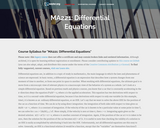Unrestricted Use
CC BY
Rating
0.0 stars

This course focuses on linear ordinary differential equations (or ODEs) and will introduce several other subclasses and their respective properties. Despite centuries of study, numerical approximation is the only practical approach to the solution of complicated ODEs that has emerged; this course will introduce you to the fundamentals behind numerical solutions. Upon successful completion of this course, students will be able to: Identify ordinary differential equations and their respective orders; Explain and demonstrate how differential equations are used to model certain situations; Solve first order differential equations as well as initial value problems; Solve linear differential equations with constant coefficients; Use power series to find solutions of linear differential equations, Solve linear systems of differential equations with constant coefficients; Use the Laplace transform to solve initial value problems; Use select methods of numerical approximation to find solutions to differential equations. (Mathematics 221; See also: Mechanical Engineering 003)

Subject:
Algebra
Mathematics
Material Type:
Full Course
Provider:
The Saylor Foundation
04/04/2019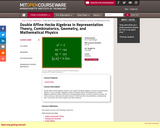Conditional Remix & Share Permitted
CC BY-NC-SA
Rating
0.0 stars

" Double affine Hecke algebras (DAHA), also called Cherednik algebras, and their representations appear in many contexts: integrable systems (Calogero-Moser and Ruijsenaars models), algebraic geometry (Hilbert schemes), orthogonal polynomials, Lie theory, quantum groups, etc. In this course we will review the basic theory of DAHA and their representations, emphasizing their connections with other subjects and open problems."

Subject:
Algebra
Geometry
Mathematics
Material Type:
Full Course
Provider:
M.I.T.
Provider Set:
M.I.T. OpenCourseWare
Author:
Etingof, Pavel
01/01/2009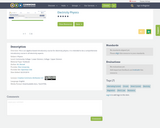Unrestricted Use
CC BY
Rating
0.0 stars

This is an algebra-based introductory course for electricity physics. It is intended to be a comprehensive introductory course in all electricity aspects, such as Ohm's Law, AC and DC Circuits, etc.

Subject:
Algebra
Applied Science
Engineering
Mathematics
Physical Science
Physics
Material Type:
Textbook
Author:
03/13/2019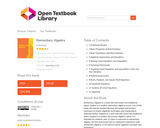Unrestricted Use
CC BY
Rating
0.0 stars

Elementary Algebra is a work text that covers the traditional topics studied in a modern elementary algebra course. Use of this book will help the student develop the insight and intuition necessary to master algebraic techniques and manipulative skills.Elementary Algebra is a work text that covers the traditional topics studied in a modern elementary algebra course. It is intended for students who (1) have no exposure to elementary algebra, (2) have previously had an unpleasant experience with elementary algebra, or (3) need to review algebraic concepts and techniques.

1 Arithmetic Review
2 Basic Properties of Real Numbers
3 Basic Operations with Real Numbers
4 Algebraic Expressions and Equations
5 Solving Linear Equations and Inequalities
6 Factoring Polynomials
7 Graphing Linear Equations and Inequalities in One and Two Variables
8 Rational Expressions
9 Roots, Radicals, and Square Root Equations
11 Systems of Linear Equations
12 Appendix

Subject:
Algebra
Mathematics
Material Type:
Textbook
Author:
Denny Burzynski
06/26/2020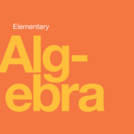Unrestricted Use
CC BY
Rating
0.0 stars

Elementary Algebra is designed to meet the scope and sequence requirements of a one-semester elementary algebra course. The book’s organization makes it easy to adapt to a variety of course syllabi. The text expands on the fundamental concepts of algebra while addressing the needs of students with diverse backgrounds and learning styles. Each topic builds upon previously developed material to demonstrate the cohesiveness and structure of mathematics.

1. Foundations
2. Solving Linear Equations and Inequalities
3. Math Models
4. Graphs
5. Systems of Linear Equations
6. Polynomials
7. Factoring
8. Rational Expressions and Equations

Access also available here: https://openstax.org/details/books/elementary-algebra

Subject:
Algebra
Mathematics
Material Type:
Textbook
Author:
MaryAnne Anthony-Smith
Lynn Marecek
03/28/2019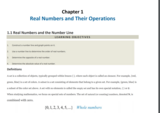Conditional Remix & Share Permitted
CC BY-NC-SA
Rating
0.0 stars

This text respects the traditional approaches to algebra pedagogy while enhancing it with the technology available today. In addition, textual notation is introduced as a means to communicate solutions electronically throughout the text. While it is important to obtain the skills to solve problems correctly, it is just as important to communicate those solutions with others effectively in the modern era of instant communications.While algebra is one of the most diversely applied subjects, students often find it to be one of the more difficult hurdles in their education. With this in mind, John wrote Elementary Algebra from the ground up in an open and modular format, allowing the instructor to modify it and leverage their individual expertise as a means to maximize the student experience and success. Elementary Algebra takes the best of the traditional, practice-driven algebra texts and combines it with modern amenities to influence learning, like online/inline video solutions, as well as, other media driven features that only a free online text can deliver.

Chapter 1: Real Numbers and Their Operations
Chapter 2: Linear Equations and Inequalities
Chapter 3: Graphing Lines
Chapter 4: Solving Linear Systems
Chapter 5: Polynomials and Their Operations
Chapter 6: Factoring and Solving by Factoring
Chapter 7: Rational Expressions and Equations
Chapter 8: Radical Expressions and Equations
Chapter 9: Solving Quadratic Equations and Graphing Parabolas
Chapter 10: Appendix: Geometric Figures

Subject:
Algebra
Mathematics
Material Type:
Textbook
Provider:
The Saylor Foundation
Provider Set:
Saylor Textbooks
Author:
John Redden
01/01/2011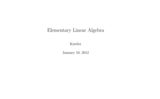Unrestricted Use
CC BY
Rating
0.0 stars

Elementary Linear Algebra was written and submitted to the Open Textbook Challenge by Dr. Kenneth Kuttler of Brigham Young University. Dr. Kuttler wrote this textbook for use by his students at BYU. According to the introduction of Elementary Linear Algebra, ĺÎĺ_ĺĚĄ_this is intended to be a first course in linear algebra for students who are sophomores or juniors who have had a course in one variable calculus and a reasonable background in college algebra.ĺÎĺ_ĺĚĺÎĺ A solutions manual for the textbook is included.

Subject:
Algebra
Mathematics
Material Type:
Textbook
Provider:
The Saylor Foundation
Provider Set:
Saylor Textbooks
Author:
Kenneth Kuttler
04/24/2019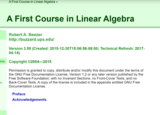Conditional Remix & Share Permitted
CC BY-SA
Rating
0.0 stars

A First Course in Linear Algebra is an introductory textbook aimed at college-level sophomores and juniors. Typically students will have taken calculus, but it is not a prerequisite. The book begins with systems of linear equations, then covers matrix algebra, before taking up finite-dimensional vector spaces in full generality. The final chapter covers matrix representations of linear transformations, through diagonalization, change of basis and Jordan canonical form. Determinants and eigenvalues are covered along the way.

Systems of Linear Equations
Vectors
Matrices
Vector Spaces
Determinants
Eigenvalues
Linear Transformations
Representations
Preliminaries
Reference

Access also available here: http://linear.ups.edu/

Subject:
Algebra
Mathematics
Material Type:
Textbook
Provider:
University of Puget Sound
Author:
Robert Beezer
04/24/2019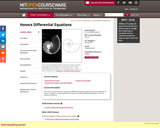Conditional Remix & Share Permitted
CC BY-NC-SA
Rating
0.0 stars

"This course covers the same material as Differential Equations (18.03) with more emphasis on theory. In addition, it treats mathematical aspects of ordinary differential equations such as existence theorems."

Subject:
Algebra
Mathematics
Material Type:
Full Course
Provider:
M.I.T.
Provider Set:
M.I.T. OpenCourseWare
Author:
Hur, Vera Mikyoung
01/01/2009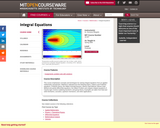Conditional Remix & Share Permitted
CC BY-NC-SA
Rating
0.0 stars

Selection of material from the following topics: calculus of variations (the first variation and the second variation); integral equations (Volterra equations; Fredholm equations, the Hilbert-Schmidt theorem); the Hilbert Problem and singular integral equations of Cauchy type; Wiener-Hopf Method and partial differential equations; Wiener-Hopf Method and integral equations; group theory.

Subject:
Algebra
Mathematics
Material Type:
Full Course
Provider:
M.I.T.
Provider Set:
M.I.T. OpenCourseWare
Author:
Margetis, Dionisios
01/01/2006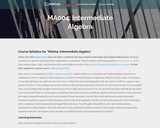Unrestricted Use
CC BY
Rating
0.0 stars

This course is a continuation of MA001: Beginning Algebra, and will focus on compound inequalities, systems of linear equations, radicals, rational exponents, quadratic equations and techniques used to solve these equations, and finally, general functions and graphs with an emphasis on the exponential and logarithmic functions.

Subject:
Algebra
Mathematics
Material Type:
Full Course
Provider:
The Saylor Foundation
04/29/2019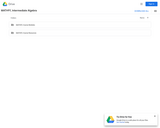Unrestricted Use
CC BY
Rating
0.0 stars

Students will learn to solve compound inequalities, absolute value inequalities, and systems of equations, simplify radical expressions, solve quadratic equations and applications and simplify compound fractions, solve rational equations and applications, use function notation to solve problems and use exponential and logarithmic functions.Login: guest_oclPassword: ocl

Subject:
Algebra
Mathematics
Material Type:
Full Course
Homework/Assignment
Lecture Notes
Syllabus
Provider:
Washington State Board for Community & Technical Colleges
Provider Set:
Open Course Library
10/31/2011Conditional Remix & Share Permitted
CC BY-NC-SA
Rating
0.0 stars

A community college level textbook that provides non-STEM students with a high-quality and academically rigorous book.

Subject:
Algebra
Mathematics
Material Type:
Textbook
Provider:
Brookdale Community College
Author:
Barbara Tozzi
Linda Wang